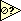# Numeric

Jump to: navigation, search

## NaN equivalent for integer data types

NaN is defined only for floating point numbers. In a U32 all the possible values are actual numbers and there is no place left for a special value that says there is nothing there. If you need an "empty" value in an integer data type you have to pick a value that never appears in your particular application (if there really is one) to serve as a flag. In other words there is no "empty value" in integer data types.

A useful trick: In most cases, zero or -1 are used as the magic sentinel value for integer work. But sometimes you'd like to use "largest possible integer" as your sentinel value because zero and -1 are actual values, and you don't expect to ever actually use the large end of the spectrum. But nobody actually remembers the largest possible integer, and even if you look it up (it is in the Context Help for an integer wire, by the way), typing it is annoying. Solution:

• Drop a Numeric constant on the block diagram and set it to be your type of integer. Then type a long string of 9s into it, such as "99999999999999999999999999999999999999999". LabVIEW will automatically change this to be the largest possible integer storable in your data type. If it doesn't convert and you still have the long string of 9s, then you didn't type enough 9s. This can happen if you're using unsigned 64-bit integers... those things are HUGE!
• Another way to get the maximum value is the following snippet:

Test a floating point wire for NaN by usingfrom the Comparison pallete.

## Comparing real numbers

What you need is a comparison that assumes that equal is the same as close enough. One way is to define an epsilon and check to make sure that your error is greater than that epsilon where epsilon is dependent on the type of number (single, double, extended) that you are using. For example, to compare A greater than or equal to B (as double precision) you could use as your test the following:

A-B >= -1e-17*(A+B)/2

Or maybe 1e-16 to be safe. To be really careful use 2^-31 as your criterion. This handles the case where A is already 10^-31 in magnitude or when both variables are zero.

A simpler method is to multiply your numbers by 10 to make integers, round them and then compare. In fact you can multiply by whatever power of 10 desired.

This is *not* a bug. It appears as a result of the limitation of finite computers. There is not now and never will be a fix. The main fix is to the programmer. As a caveat, do not ever, ever, ever compare real numbers. They are uncertain within the least significant bit.

These uncertainties arise in several situations. Another example where this must be taken into account is the output of both"Format & Strip" and "From Exponential/Fract/Eng"; enter a string of "0.1" and you get the number: 0.10000000000000000100. You cannot represent 0.1 as a finite binary string.

As to a wish for a finite conversion width, nope. The problem is that the conversion does have a finite width. It is that factor of 5 in base 10 numbers that makes a finite decimal be an infinite repeating binary string. You can represent all numbers that can be formed as sums of 1/2, 1/4, 1/8, 1/16, 1/32. Unfortunately 1/10 can only be approximated. The wish seems to indicate that the binary is converted to base 10 (with a finite conversion width) and then compared. Actually it is all compared in binary, when you display it, you can convert it to 10 digits or what ever you want.

In summary, any binary representation will be approximate. How approximate is based on the word length. It is that factor of 5 in base 10 numbers that gets us. It appears that you have 17 digits of accuracy which is about right for a double precision number. Extended have more, singles have less. That is mathematical reality. Computers are not idealized mathematical machines. If it was easy everyone would be an expert. Knowing how things are treated by the underlying hardware can keep one from having these problems.